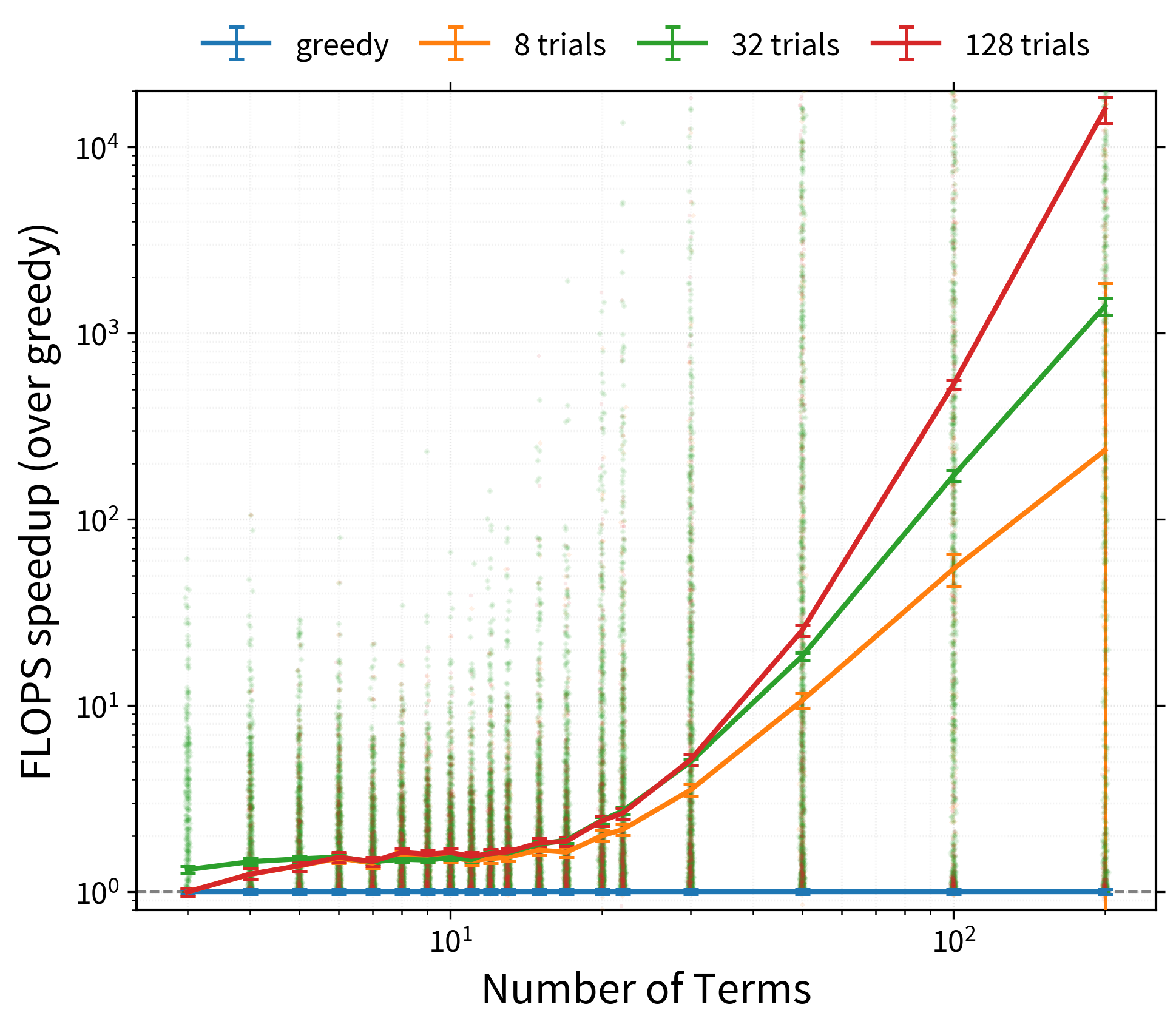# The Random-Greedy Path

For large and complex contractions the exhaustive approaches will be too slow while the greedy path might be very far from optimal. In this case you might want to consider the 'random-greedy' path optimizer. This samples many greedy paths and selects the best one found, which can often be exponentially better than the average.

import opt_einsum as oe
import numpy as np
import math

eq, shapes = oe.helpers.rand_equation(40, 5, seed=1, d_max=2)
arrays = list(map(np.ones, shapes))

path_greedy = oe.contract_path(eq, *arrays, optimize='greedy')
print(math.log2(path_greedy.opt_cost))
#> 36.04683022558587

path_rand_greedy = oe.contract_path(eq, *arrays, optimize='random-greedy')
print(math.log2(path_rand_greedy.opt_cost))
#> 32.203616699170865


So here the random-greedy approach has found a path about 16 times quicker (= 2^(36 - 32)).

This approach works by randomly choosing from the best n contractions at each step, weighted by a Boltzmann factor with respect to the contraction with the 'best' cost. As such, contractions with very similar costs will be explored with equal probability, whereas those with higher costs will be less likely, but still possible. In this way, the optimizer can randomly explore the huge space of possible paths, but in a guided manner.

The following graph roughly demonstrates the potential benefits of the 'random-greedy' algorithm, here for large randomly generated contractions, with either 8, 32 (the default), or 128 repeats:Note

Bear in mind that such speed-ups are not guaranteed - it very much depends on how structured or complex your contractions are.

## Customizing the Random-Greedy Path

The random-greedy optimizer can be customized by instantiating your own opt_einsum.paths.RandomGreedy object. Here you can control:

• temperature - how far to stray from the locally 'best' contractions
• rel_temperature - whether to normalize the temperature
• nbranch - how many contractions (branches) to consider at each step
• cost_fn - how to cost potential contractions

There are also the main opt_einsum.paths.RandomOptimizer options:

• max_repeats - the maximum number of repeats
• max_time - the maximum amount of time to run for (in seconds)
• minimize - whether to minimize for total 'flops' or 'size' of the largest intermediate

For example, here we'll create an optimizer, then change its temperature whilst reusing it. We'll also set a high max_repeats and instead use a maximum time to terminate the search:

optimizer = oe.RandomGreedy(max_time=2, max_repeats=1_000_000)

for T in [1000, 100, 10, 1, 0.1]:
optimizer.temperature = T
path_rand_greedy = oe.contract_path(eq, *arrays, optimize=optimizer)
print(math.log2(optimizer.best['flops']))

#> 32.81709395639357
#> 32.67625007170783
#> 31.719756871539033
#> 31.62043317835677
#> 31.253305891247

# the total number of trials so far
print(len(optimizer.costs))
#> 2555


So we have improved a bit on the standard 'random-greedy' (which performs 32 repeats by default). The optimizer object now stores both the best path found so far - optimizer.path - as well as the list of flop-costs and maximum sizes found for each trial - optimizer.costs and optimizer.sizes respectively.

Since each greedy attempt is independent, the random-greedy approach is naturally suited to parallelization. This can be automatically handled by specifying the parallel keyword like so:

# use same number of processes as cores
optimizer = oe.RandomGreedy(parallel=True)

# or use specific number of processes
optimizer = oe.RandomGreedy(parallel=4)


Warning

The pool-executor used to perform this parallelization is the ProcessPoolExecutor from the concurrent.futures module.

For full control over the parallelization you can supply any pool-executor like object, which should have an API matching the Python 3 concurrent.futures module:

from concurrent.futures import ProcessPoolExecutor

pool = ProcessPoolExecutor()
optimizer = oe.RandomGreedy(parallel=pool, max_repeats=128)
path_rand_greedy = oe.contract_path(eq, *arrays, optimize=optimizer)

print(math.log2(optimizer.best['flops']))
#> 31.64992600300931


Other examples of such pools include: